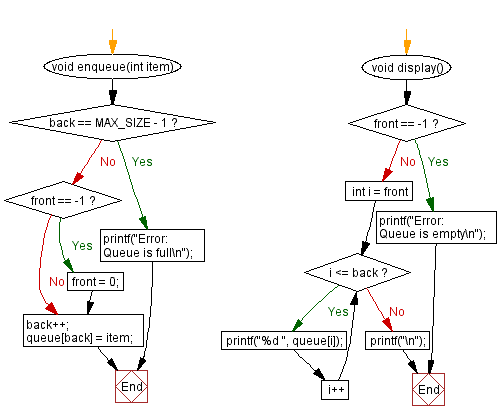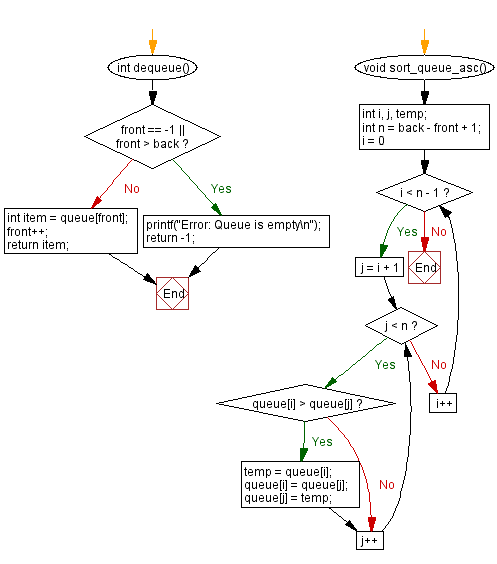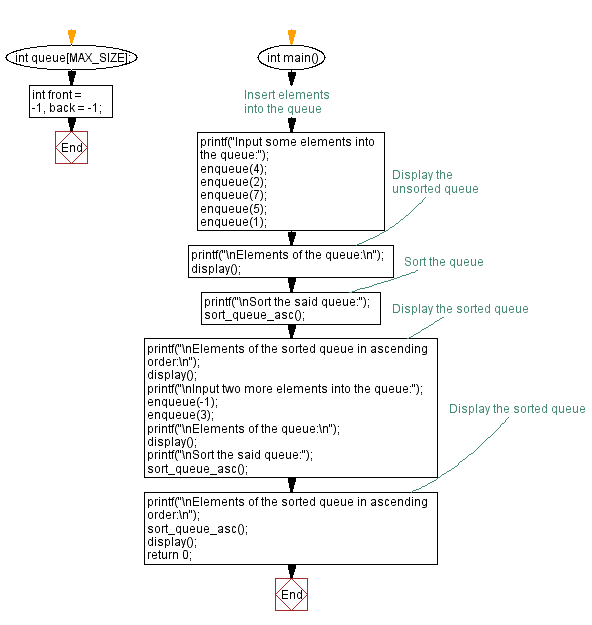﻿ C - Sort a queue in ascending order

# C Exercises: Sort a queue in ascending order

## C Queue: Exercise-12 with Solution

Write a C program to sort the elements of a queue in ascending order.

Sample Solution:

C Code:

``````#include <stdio.h>

#define MAX_SIZE 100
int queue[MAX_SIZE];
int front = -1, back = -1;
void enqueue(int item) {
if (back == MAX_SIZE - 1) {
printf("Error: Queue is full\n");
return;
}
if (front == -1) {
front = 0;
}
back++;
queue[back] = item;
}
int dequeue() {
if (front == -1 || front > back) {
printf("Error: Queue is empty\n");
return -1;
}
int item = queue[front];
front++;
return item;
}
void display() {
if (front == -1) {
printf("Error: Queue is empty\n");
return;
}
for (int i = front; i <= back; i++) {
printf("%d ", queue[i]);
}
printf("\n");
}
void sort_queue_asc() {
int i, j, temp;
int n = back - front + 1;

for (i = 0; i < n - 1; i++) {
for (j = i + 1; j < n; j++) {
if (queue[i] > queue[j]) {
temp = queue[i];
queue[i] = queue[j];
queue[j] = temp;
}
}
}
}
int main() {
// Insert elements into the queue
printf("Input some elements into the queue:");
enqueue(4);
enqueue(2);
enqueue(7);
enqueue(5);
enqueue(1);
// Display the unsorted queue
printf("\nElements of the queue:\n");
display();
// Sort the queue
printf("\nSort the said queue:");
sort_queue_asc();
// Display the sorted queue
printf("\nElements of the sorted queue in ascending order:\n");
display();
printf("\nInput two more elements into the queue:");
enqueue(-1);
enqueue(3);
printf("\nElements of the queue:\n");
display();
printf("\nSort the said queue:");
sort_queue_asc();
// Display the sorted queue
printf("\nElements of the sorted queue in ascending order:\n");
sort_queue_asc();
display();
return 0;
}
```
```

Sample Output:

```Input some elements into the queue:
Elements of the queue:
4 2 7 5 1

Sort the said queue:
Elements of the sorted queue in ascending order:
1 2 4 5 7

Input two more elements into the queue:
Elements of the queue:
1 2 4 5 7 -1 3

Sort the said queue:
Elements of the sorted queue in ascending order:
-1 1 2 3 4 5 7
```

Flowchart:C Programming Code Editor:

Have another way to solve this solution? Contribute your code (and comments) through Disqus.

What is the difficulty level of this exercise?

Test your Programming skills with w3resource's quiz.

﻿

## C Programming: Tips of the Day

C Programming - How do you pass a function as a parameter in C?

Declaration

A prototype for a function which takes a function parameter looks like the following:

```void func ( void (*f)(int) );
```

This states that the parameter f will be a pointer to a function which has a void return type and which takes a single int parameter. The following function (print) is an example of a function which could be passed to func as a parameter because it is the proper type:

```void print ( int x ) {
printf("%d\n", x);
}
```

Function Call

When calling a function with a function parameter, the value passed must be a pointer to a function. Use the function's name (without parentheses) for this:

```func(print);
```

would call func, passing the print function to it.

Function Body

As with any parameter, func can now use the parameter's name in the function body to access the value of the parameter. Let's say that func will apply the function it is passed to the numbers 0-4. Consider, first, what the loop would look like to call print directly:

```for ( int ctr = 0 ; ctr < 5 ; ctr++ ) {
print(ctr);
}
```

Since func's parameter declaration says that f is the name for a pointer to the desired function, we recall first that if f is a pointer then *f is the thing that f points to (i.e. the function print in this case). As a result, just replace every occurrence of print in the loop above with *f:

```void func ( void (*f)(int) ) {
for ( int ctr = 0 ; ctr < 5 ; ctr++ ) {
(*f)(ctr);
}
}
```

Ref : https://bit.ly/3skw9Um